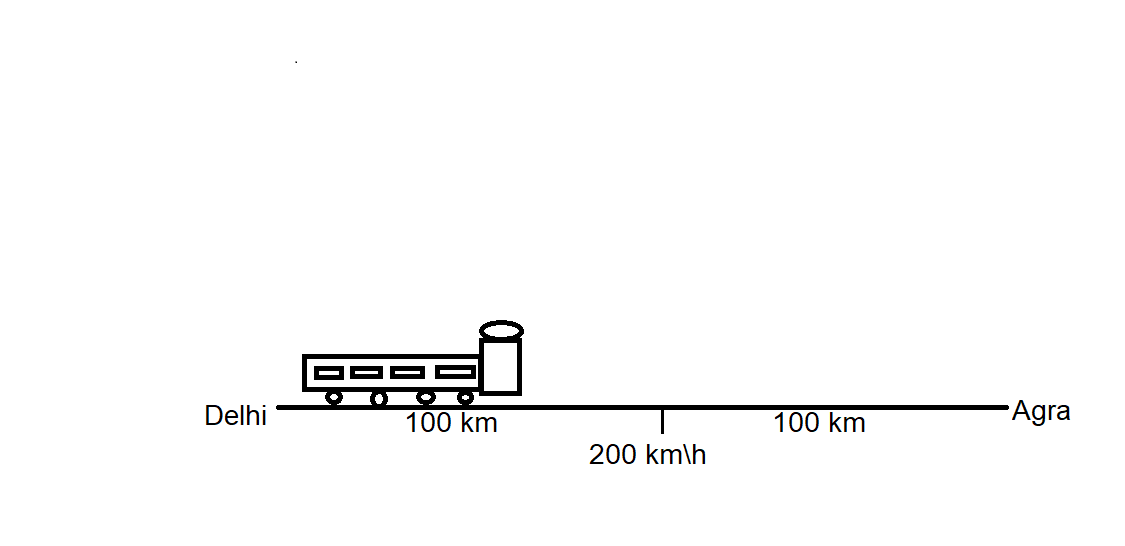# Distance between Delhi and Agra is 200 km. A train travels the first 100 km at a speed of 50 km/hr how fast must the train travel the next hundred kilometre so as to average 70km/hr for the whole journey?For the first $100\ km$:

Distance$=100\ km$

Speed$=50\ km/h$

Therefore, Time taken for first part of journey $t_2=\frac{Distance}{Speed}=\frac{100}{50}=2\ h$

For the second part of journey:

Let $s\ km/h$ be the speed of the train for the second part of the journey:

Distance$=100\ km$

Time taken for the second part of journey $t_2=\frac{Distance}{Speed}=\frac{100}{s}$

Now, as we know that:

$Average\ speed=\frac{Total\ distance\ traveled}{Total\ time\ taken}$

Where, Average speed$=70\ km/h$

Total distance travelled$=200\ km$

Total time taken $t=t_1+t_2=2+\frac{100}{s}$

On substituting these values in the average speed equation, we get:

Average speed$=\frac{Total\ distance\ traveled}{Total\ time\ taken}$

or, $70=\frac{200}{( 2 + \frac{100}{s})}$

or, $70\times( 2 + \frac{100}{s})=200$

or, $140 + \frac{7000}{s}=200$

or, $\frac{7000}{s}=60$

or, $s=\frac{7000}{60}=116.67\ km/h$

Therefore, the speed of the train must be $116.67\ km/h$ for the average speed of $70\ km/h$ for the whole journey.

Updated on: 10-Oct-2022

247 Views